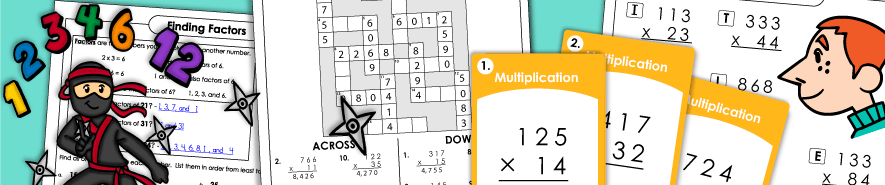# Multiplication Worksheets (Multi-Digit)## Multi-Digit Multiplication

Multiplication: 2 Digits Times 1 Digit

Here you'll discover a large selection of games, worksheets, and task cards for practicing 2-digit by 1-digit multiplication. (example: 51x3)

Multiplication: 3 Digits Times 1 Digit

On this page you'll have a large selection of worksheets and games for multiplying 3-digit by 1-digit numbers. (example: 929x6)

Multiplication: 4 Digits Times 1 Digit

Practice finding the products of 4-digit numbers and 1-digit numbers. (example: 4,527x9)

Multiplication: 2 Digits Times 2 Digits

These printables have pairs of double digit numbers for students to multiply together. (example: 35x76)

Multiplication: 3 Digits Times 2 Digits

On these exercises, students will find the products of 3-digit and 2-digit numbers.

Multiplication by 3-Digit Numbers

Lattice Multiplication

Use lattice grids to multiply multi-digit numbers together. Includes activities with explanations, as well as blank practice grids.

## Multiplying Money, Decimals, and Fractions

Multiplying Money

Browse our collection of worksheets, task cards, and games for multiplying money.

Multiplying Decimals

Multiply pairs of decimal numbers together.

Multiplying Fractions

With these files, students can review multiplying pairs of fractions and mixed numbers.

## Worksheet Generator

Multi-Digit Multiplication Generator

Use this tool to make your own multi-digit multiplication printables. You can toggle between horizontal and vertical problems, as well as choose the number of digits in each factor.

## Multiples and Factors

Factors and GCF

Find factors all of a number, as well as the greatest common factor.

Multiples

With these printables, you can teach students to identify multiples of numbers.

Least Common Multiple

Learn about least common multiple with these resources.

## Basic Multiplication (Single Digit)

Basic Multiplication (0-10)

Practice basic multiplication facts that go up to 10x10.

Basic Multiplication (0-12)

Review and practice basic facts up to 12x12. Includes a variety of fun games, timed quizzes, drill worksheets, task cards, fact families, flashcards, and puzzles.